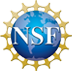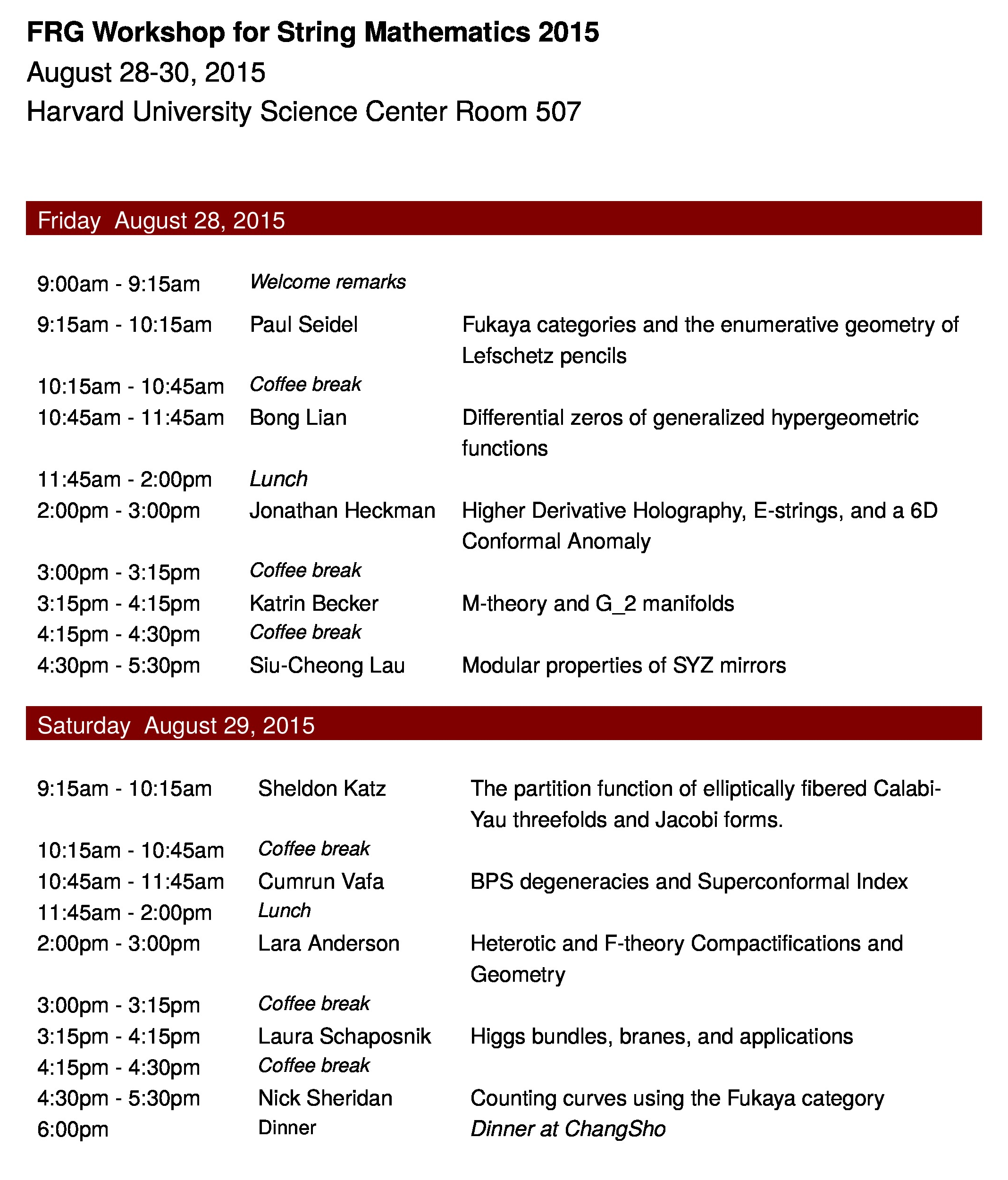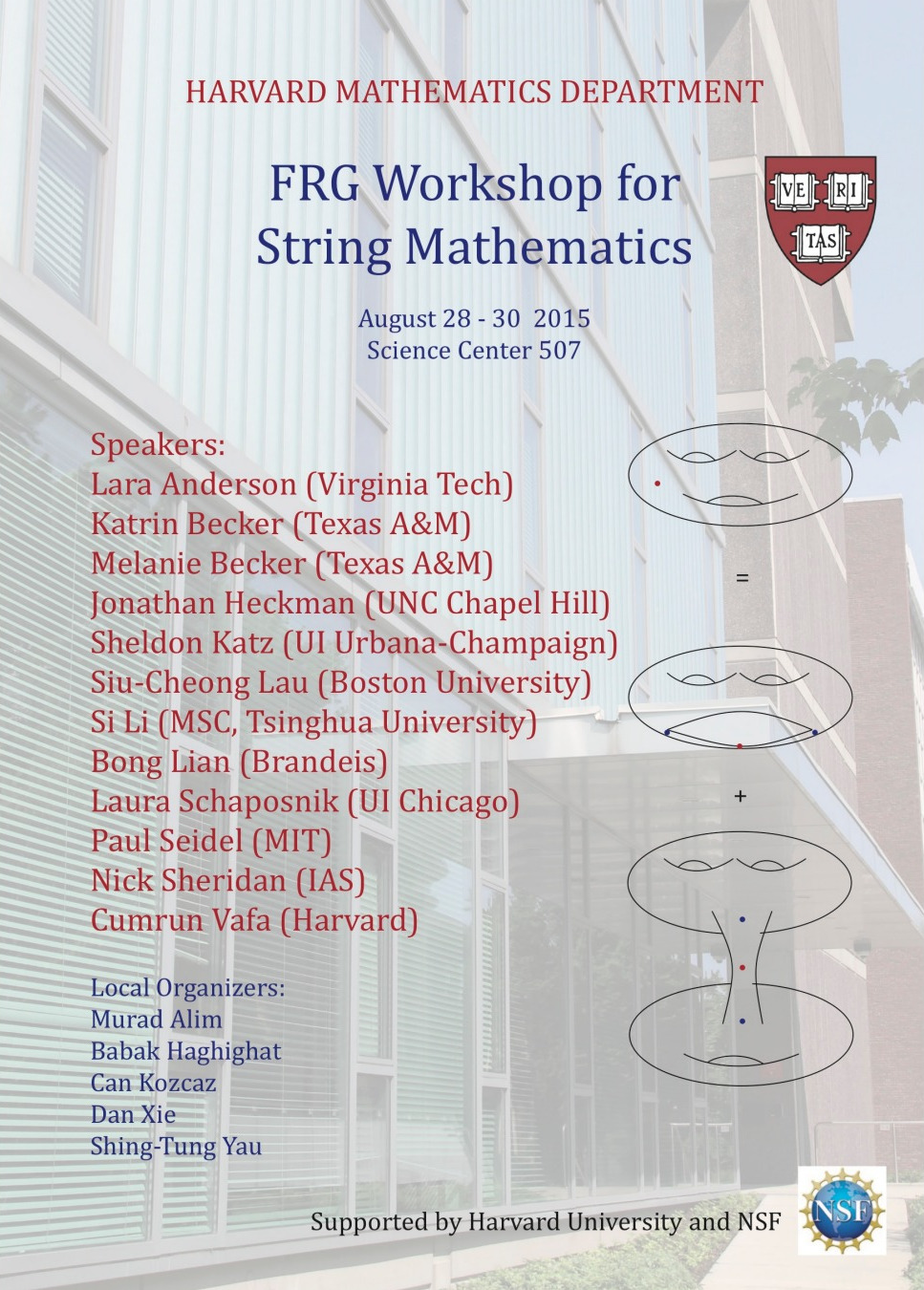## FRG Workshop in Cambridge

#### August 28-29, 2015, Harvard University, Science Center Room 507This is a NSF funded Focused Research Groups FRG`

#### Talk titles and abstracts:

 Lara Anderson. Heterotic and F-theory Compactifications and Geometry We systematically analyze a broad class of dual heterotic and F-theory models that give rise to six- and four-dimensional supergravity theories, and compare the geometric constraints on the two sides of the duality. In this talk we will demonstrate that both theories together give new insight into the space of possible effective theories arising from string theory. We will describe recent explicit constructions of all dual F-theory/heterotic pairs in a broad class and discuss aspects of G-flux in F-theory. Katrin Becker. M-theory and G_2 manifolds This is an introduction to M-theory which is a theory of quantum gravity. It unifies general relativity with quantum field theory. M-theory includes besides all string theories eleven-dimensional supergravity. In a Kaluza and Klein approach to unification, a four-dimensional theory can be obtained by assuming the additional seven dimensions to be small and compact. Unbroken supersymmetry requires that the seven dimensional manifold admits a G2 holonomy metric. We will discuss how these classical solutions become solutions of the quantum theory. Jonathan Heckman. Higher Derivative Holography, E-strings, and a 6D Conformal Anomaly In this talk we show how to use a recently proposed formula for the conformal anomaly a_{6D} of 6D superconformal field theories to probe the structure of higher derivative corrections to 11D supergravity in the presence of non-trivial four-form fluxes and a Horava-Witten 9-brane. Sheldon Katz. The partition function of elliptically fibered Calabi-Yau threefolds and Jacobi forms I give evidence that the all genus amplitudes of topological string theory on compact elliptically fibered Calabi-Yau manifolds can be written in terms of meromorphic Jacobi forms whose weight grows linearly and whose index grows quadratically with the base degree. This talk is based on joint work with Albrecht Klemm and Minxin Huang. Siu-Cheong Lau. Modular properties of SYZ mirrors In this talk we will construct the SYZ mirrors of certain infinite-type toric Calabi-Yau manifolds and their quotients, and investigate their properties under modular transformations of the global moduli. We will also study the generalized SYZ mirrors of elliptic curve quotients. While an SYZ mirror is a priori defined over a formal neighborhood around the large volume limit, the study in all these cases suggests that it is indeed the tip of the iceberg over the global moduli space. Bong Lian. Differential zeros of generalized hypergeometric functions I will discuss a new way to compute zero locus of certain differential polynomials of period integrals of families of algebraic varieties, including Calabi-Yau and general type hypersurfaces in a G-space. These zero locus generalizes zeros of classical hypergeometric functions in one variable. The method is based on a new algebraic description of Picard-Fuchs systems for the period integrals, and generalized hypergeometric functions in particular. The talk is based on joint work with J. Chen, A. Huang, S.-T. Yau, and X. Zhu. Laura Schaposnik. Higgs bundles, branes, and applications. Higgs bundles (introduced by N. Hitchin in 1987) are pairs of holomorphic vector bundles and holomorphic 1-forms taking values in the endomorphisms of the bundle. The moduli space of Higgs bundles carries a natural Hyperkahler structure, through which we can study A-branes (Lagrangian subspaces) or B-branes (holomorphic subspaces) with respect to each structure. We shall begin the talk by first introducing Higgs bundles for complex Lie groups and the associated Hitchin fibration, and recalling how to realize Langlands duality through spectral data. We will then look at a natural construction of families of subspaces which give different types of branes, and explain how the topology of some of these branes can be completely determined via the monodromy action of the Hitchin system. Finally, we shall give some applications of the above approaches in relation to Langlands duality and the study of character varieties. Some of the work presented during the talk is in collaboration with David Baraglia (Adelaide). Paul Seidel. Fukaya categories and the enumerative geometry of Lefschetz pencils I will describe the conjectural interplay between Fukaya categories of Lefschez fibrations and mirror maps for Calabi-Yau hypersurfaces. Nick Sheridan. Counting curves using the Fukaya category In 1991, string theorists Candelas, de la Ossa, Green and Parkes made a startling prediction for the number of curves in each degree on a generic quintic threefold, in terms of periods of a holomorphic volume form on a `mirror manifold'. Givental and Lian, Liu and Yau gave a mathematical proof of this version of mirror symmetry for the quintic threefold (and many more examples) in 1996. In the meantime (1994), Kontsevich had introduced his `homological mirror symmetry' conjecture and stated that it would `unveil the mystery of mirror symmetry'. I will explain how to prove that the number of curves on the quintic threefold matches up with the periods of the mirror via homological mirror symmetry. I will also attempt to explain in what sense this is `less mysterious' than the previous proof. Cumrun Vafa. BPS degeneracies and Superconformal Index. Abstract: I will discuss the relation between BPS degeneracies and specialization of superconformal index in 2 and 4 dimensions and its relation with wall-crossing phenomena.Funded by NSF and Harvard Mathematics Department. Last update: July 10, 2015